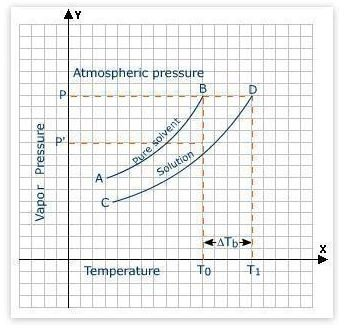# Which Boils or Freezes Faster, Salt or Sugar Water? The Technical ExplanationThe colligative properties of solutions depend upon the number of molecules dissolved in the solution, but not on the type of solutes. Pure water freezes at 32 F and boils at 212 F. However, adding any kind of solute to pure water will lower the freezing point.

For example, salt water has a lower freezing point than that of pure water. Here you should note that if the molar amounts of two different solutes are same and the number of ions present in the solution are same, it does not matter which kind of salt you are putting into the water; the freezing point or for that matter, the boiling point, will be same. The boiling point elevation and freezing point depression are the colligative properties.

## Boiling Times

If the number of number of sugar and salt moles are same in their respective solutions and the heating rate is same, salt water would boil faster than sugar water.

You can understand this process better if you look at the equation

Δ T b = i K b m

where Δ T b is the change in boiling point, K b is the boiling point elevation constant for the solvent that is 0.52°C•kg/mol for water, m is the molality of solution that is moles solute /kg solvent, and i is the number of ions present per formula unit. There are 2 ions for each NaCl molecule.

Now, if we add the same mass of NaCl and sucrose to the same mass of water, the NaCl will have higher moles than that of sugar, since mw of NaCl is 58.4 g/mole and for sucrose, it is 342 g/mole. Moreover, each salt molecule splits into two ions, a sodium atom and a chloride atom, increasing the number of particles present in the water.

For instance, adding 10 g of salt into 100 g of water will add approximately 6 times more particles than if you add 10 g sucrose to the equal mass of water. Moreover, sugar molecules do not split further. Thus, adding the same mass of sugar into the same amount of water will add far fewer particles than that of salt. It results in lower Δ T b for salt water and hence, a lower boiling point for salt water as compared to sugar water.

## Freezing Times

No. Salt water has a lower freezing point than that of sugar water; it will take more time to freeze. The presence of a solute lowers the freezing point when compared to pure water. This phenomenon can also be explained by the colligative property of solutions. As explained above, if you add same masses of salt and sugar in equal masses of water, the salt solution will have more particles. It will result in a greater lowering of the freezing point in a salt solution than in a sugar solution.

You can check this using the given formula.

Δ T f = i K f m

Where Δ T f is the change in the freezing point, K f is the freezing depression constant for the solvent, and i and m are the same as in boiling point formula explained above.

## Conclusion

Salt water boils faster than sugar water. On the other hand, sugar water freezes faster than salt water.